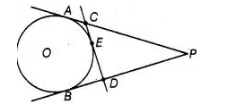# From an external point P, two tangents,

Question:

From an external point P, two tangents, PA and PB are drawn to a circle with centre 0. At one point E on the circle tangent is drawn which intersects PA

and PB at C and D, respectively. If PA = 10 cm, find the perimeter of the trianlge PCD.

Solution:

Two tangents PA and PB are drawn to a circle with centre 0 from an external point PPerimeter of $\triangle P C D=P C+C D+P D$

$=P C+C E+E D+P D$

$=P C+C A+D B+P D$

$=P A+P B$

$=2 P A=2(10)$

$=20 \mathrm{~cm}$

$[\because C E=C A, D E=D B, P A=P B$ tangents from internal point to a circle are equal]